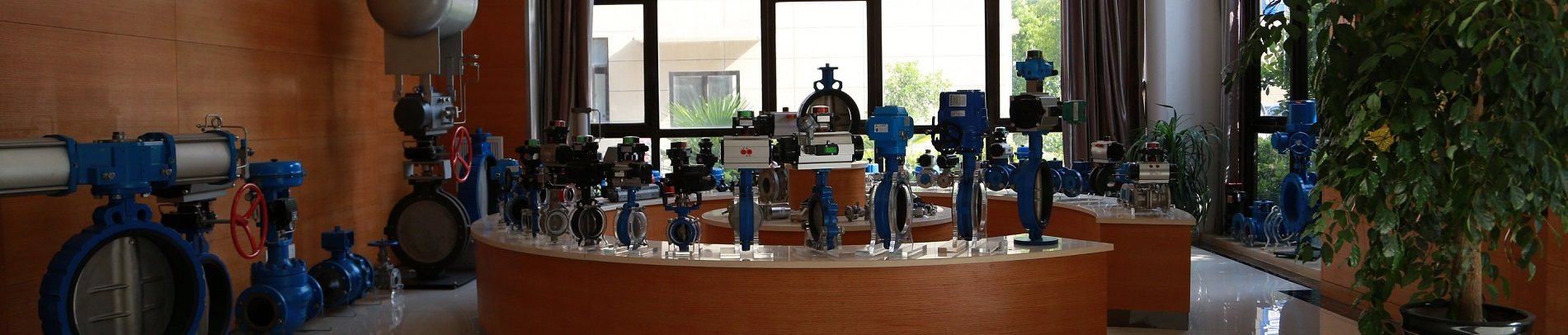# 调节阀的计算

R=电阻，单位为欧姆

V=电压降，单位为伏特

I=电流（安培）

R=流体“阻力”

P1=上游流体压力

P2=下游流体压力

Q=体积流体流量

k=湍流能量耗散系数

ρ=流体的质量密度

A=限制的横截面积

Q=液体的体积流量（加仑/分钟，GPM）

Cv=阀门的流量系数

P1=液体上游压力（PSI）

P2=液体的下游压力（PSI）

Gf=液体比重（液体密度与标准水密度之比）